# JP2008122228A - System and method for positioning - Google Patents

## Info

Publication number
JP2008122228A
JP2008122228A JP2006306406A JP2006306406A JP2008122228A JP 2008122228 A JP2008122228 A JP 2008122228A JP 2006306406 A JP2006306406 A JP 2006306406A JP 2006306406 A JP2006306406 A JP 2006306406A JP 2008122228 A JP2008122228 A JP 2008122228A
Authority
JP
Japan
Prior art keywords
means
dimensional
measuring
measurement
Prior art date
Legal status (The legal status is an assumption and is not a legal conclusion. Google has not performed a legal analysis and makes no representation as to the accuracy of the status listed.)
Pending
Application number
JP2006306406A
Other languages
Japanese (ja)
Inventor
Koji Morimoto

Original Assignee
Konica Minolta Sensing Inc
コニカミノルタセンシング株式会社
Priority date (The priority date is an assumption and is not a legal conclusion. Google has not performed a legal analysis and makes no representation as to the accuracy of the date listed.)
Filing date
Publication date
Application filed by Konica Minolta Sensing Inc, コニカミノルタセンシング株式会社 filed Critical Konica Minolta Sensing Inc
Priority to JP2006306406A priority Critical patent/JP2008122228A/en
Publication of JP2008122228A publication Critical patent/JP2008122228A/en
Application status is Pending legal-status Critical

• 239000007787 solids Substances 0 abstract 7
• 238000004364 calculation methods Methods 0 abstract 1
• 238000006243 chemical reaction Methods 0 abstract 1
• 230000015654 memory Effects 0 abstract 1

## Images

•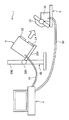•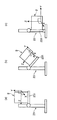•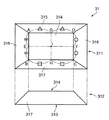•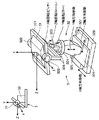•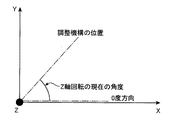•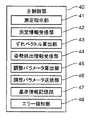•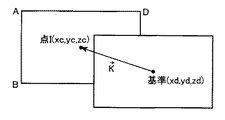•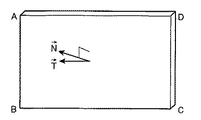•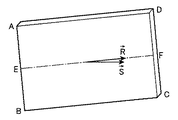•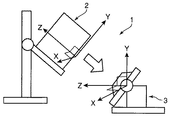•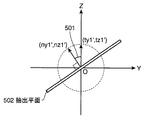•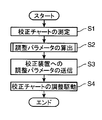•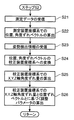•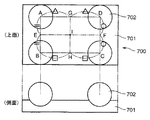•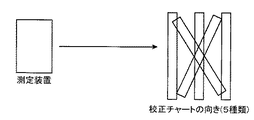•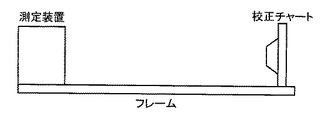•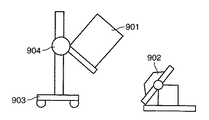## Classifications

• GPHYSICS
• G01MEASURING; TESTING
• G01BMEASURING LENGTH, THICKNESS OR SIMILAR LINEAR DIMENSIONS; MEASURING ANGLES; MEASURING AREAS; MEASURING IRREGULARITIES OF SURFACES OR CONTOURS
• G01B11/00Measuring arrangements characterised by the use of optical means
• G01B11/02Measuring arrangements characterised by the use of optical means for measuring length, width or thickness
• G01B11/03Measuring arrangements characterised by the use of optical means for measuring length, width or thickness by measuring coordinates of points

## Abstract

<P>PROBLEM TO BE SOLVED: To provide a system and a method for positioning, which can be implemented easily and with high accuracy, without requiring a large space. <P>SOLUTION: The system include a solid measuring object which is a solid measuring target; a measurement means for three dimensionally measuring the solid measuring object in a non-contact manner to acquire three dimensional data of the solid measurement object; a memory for previously storing reference information that serves as a reference in positioning between the measuring means and the solid measuring object; a deviation calculation means for calculating positional and angular deviations between the measuring means and the solid measuring object, in the coordinate system of the measuring means; a conversion means for converting the positional and angular deviations to adjustment parameters for adjustment-operation in the positioning; and an adjustment means for adjusting the position and the angle of the solid measuring object, based on the adjustment parameters. <P>COPYRIGHT: (C)2008,JPO&INPIT

## Description

The present invention relates to an alignment system and an alignment method for performing alignment between two measuring devices and an object to be measured.

Conventionally, a method for measuring a three-dimensional shape of a measurement object using a non-contact three-dimensional measuring device (hereinafter also simply referred to as “measuring device”), that is, measuring a three-dimensional shape, is an industry such as CG (Computer Graphics), design, or automobile. It is used for various applications such as The measurement principle of this three-dimensional measurement includes a light cutting method using laser slit light, a pattern projection method using pattern light, and a stereo based on images obtained by photographing the same measurement object from a plurality of different gaze directions. It is known that three-dimensional data composed of polygons or the like is obtained from measurement data obtained using a moire method or a moire method using moire.

In a non-contact three-dimensional measuring apparatus, it is important to ensure measurement accuracy (meaning of dimensional accuracy), and the measurement apparatus is calibrated at a factory or the like, and is shipped after performing an accuracy inspection. However, in the measurement environment of the user, the accuracy error of the measurement apparatus may occur due to the following factors even when the calibration or inspection is performed.
・ Expansion and contraction of mechanical members due to environmental changes in temperature and humidity, or changes in electrical characteristics ・ Distortion of mechanical members and optical systems due to changes in posture of measurement devices ・ Lens replacement (measurement devices with removable lenses) Case) That is, the optical system shifts by attaching and detaching the lens.

Therefore, it is necessary to perform calibration for correcting the accuracy error due to these in the measurement environment of the user. This calibration is called user calibration. In general, a calibration chart is measured for user calibration. A calibration chart is a measurement object for calibration whose dimensions are known. In user calibration, various calibration parameters are optimized so that the error between the dimension of the measurement object actually measured and the known dimension of the measurement object for calibration is minimized.

When performing the measurement in the user calibration, it is necessary to determine (position) the positional relationship between the measurement apparatus and the calibration chart (measurement object). The positional relationship between the measuring device and the calibration chart is determined by one or a plurality of patterns. For example, a total of 7 patterns when the calibration chart is directly opposed to the measurement device to be the near side position, the center position, and the far side position, and when the measurement device is diagonally upward, diagonally downward, diagonally rightward, and diagonally leftward The positional relationship of FIG. 15 shows the positional relationship in the case of five patterns of near side, center and far side positions, and diagonally upward and diagonally downwards.
JP 2000-97636 A

Regarding the positioning of the measuring device and the calibration chart, there is known a method of positioning by mounting (fixing) the calibration chart and the measuring device on a predetermined support member such as a frame (calibration chart frame), that is, a positioning method using a jig. It has been. However, this method has the following problems.

-If there is unevenness in the location where the calibration chart is installed, the calibration chart frame may be distorted, resulting in displacement of the installation position, resulting in poor calibration accuracy.
When the measuring device is attached to a stand or the like, it takes time to remove the measuring device from the stand and place it on the frame, and there is a risk of damage due to dropping or the like. When the measuring device is heavy, an additional burden is placed on the operator when removing and installing the measuring device.
-Since calibration is performed only in a specific posture (for example, a directly-facing posture), calibration cannot be performed in the same posture as when actually measuring. That is, a measurement error due to bending or distortion of each device member due to a different posture (posture difference; triangulation error due to a change in baseline length between the light emitting portion and the light receiving portion of the measuring device in an embodiment described later) appears in the measured value. End up.
・ If the frame is long, a large storage space is required (see FIG. 16).

Regarding the above positioning, as shown in FIG. 17, in Patent Document 1, a two-dimensional image (monitor image) of a measurement object 902 is acquired by a measuring device 901, and a user has a certain point on the two-dimensional image. That is, when the reference point is designated, the position and angle of the measuring device 901 relative to the measurement object 902 are adjusted by the position adjusting mechanism 903 and the angle adjusting mechanism 904 so that the reference point comes to the origin (center point) of the coordinate system of the measuring device. An automatically controlled technique is disclosed. However, this technique has the following problems.

The work procedure includes designation of the alignment reference point by the user (instruction to align with this position), and the alignment cannot be performed automatically.
・ A solid measurement object (measurement object) with a known shape is not assumed.
・ When calibrating with multiple positional relationships (diagonal direction, etc.), the measurement device must be moved around the calibration chart, that is, the location of the measurement device must be moved and the orientation and orientation must be changed. (Installation space or movement space) is required.
-When calibrating with a plurality of positional relationships, the measuring device is moved as described above, and therefore, calibration may not be performed in the same posture as when actually measuring (an attitude difference occurs).
-In the method of instructing a point with respect to a two-dimensional image, although it is possible to roughly align, it is difficult to align the position and angle with high accuracy.

The present invention has been made in view of the above circumstances, does not require a wide space, and can be easily aligned without requiring an operation or the like for the user to indicate a reference position. An object of the present invention is to provide an alignment system and an alignment method capable of performing alignment with high accuracy by preventing occurrence of a posture difference or the like.

The alignment system according to the present invention includes a three-dimensional measurement object that is a three-dimensional measurement object, and a measurement unit that three-dimensionally measures the three-dimensional measurement object in a non-contact manner and acquires three-dimensional data of the three-dimensional measurement object; A storage unit that stores in advance reference information serving as a reference for alignment between the measurement unit and the three-dimensional measurement object; and the measurement unit in the coordinate system of the measurement unit based on the three-dimensional data and the reference information; A deviation amount calculating means for calculating a mutual positional deviation amount and an angular deviation amount with the three-dimensional measuring object, a conversion means for converting the positional deviation amount and the angular deviation amount into adjustment parameters for an adjustment operation in the alignment, and And adjusting means for adjusting the position and angle of the three-dimensional object based on the adjustment parameter.

According to the above configuration, the three-dimensional measurement object, which is a three-dimensional measurement object, is three-dimensionally measured in a non-contact manner by the measurement unit, and three-dimensional data of the three-dimensional measurement object is acquired. In addition, the storage unit stores in advance reference information that serves as a reference for alignment between the measurement unit and the three-dimensional object. Further, the displacement amount calculating means calculates the mutual positional deviation amount and angular deviation amount between the measuring means and the three-dimensional measuring object in the coordinate system of the measuring means based on the three-dimensional data and the reference information. Then, the conversion unit converts the positional deviation amount and the angular deviation amount into adjustment parameters for adjustment operation in alignment, and the adjustment unit adjusts the position and angle of the three-dimensional object based on the adjustment parameters.

Further, in the above configuration, the apparatus further includes an attitude detection unit that detects an attitude of the three-dimensional measurement object, and the conversion unit uses the attitude detection information obtained by the attitude detection unit and uses the coordinate system of the measurement unit and the coordinates of the adjustment unit. By calculating a relational expression indicating the relationship with the system and performing coordinate transformation based on the relational expression, the positional deviation amount and the angular deviation amount in the coordinate system of the measuring means are converted into the adjustment parameter in the coordinate system of the adjusting means. You may make it convert into. (Claim 2)

According to this, the posture of the three-dimensional object is detected by the posture detection means. Then, the conversion means calculates the relational expression indicating the relationship between the coordinate system of the measurement means and the coordinate system of the adjustment means using the posture detection information by the posture detection means, and performs coordinate conversion based on the relational expression. The positional deviation amount and the angular deviation amount in the coordinate system of the measuring means are converted into adjustment parameters in the coordinate system of the adjusting means.

Further, in the above configuration, the three-dimensional object is a reference three-dimensional object having a known shape for calibrating the measuring unit, and the adjusting unit performs the alignment as a stage before performing the calibration. You may make it adjust the position and angle of the said reference | standard solid object in order to perform. (Claim 3)

According to this, the three-dimensional object is a reference three-dimensional object with a known shape for calibrating the measuring means. Then, the position and angle of the reference three-dimensional object are adjusted by the adjusting means so as to perform alignment as a previous stage of calibration.

In the above configuration, the adjustment unit may include an automatic adjustment mechanism that is automatically driven based on the adjustment parameter to perform the adjustment. (Claim 4)

Further, in the above configuration, adjustment information notification means for notifying a user of information relating to the adjustment parameter is further provided, and the adjustment means includes a manual adjustment mechanism that is driven by the user's manual operation to perform the adjustment. Also good. (Claim 5)

According to this, the information regarding the adjustment parameter is notified to the user by the adjustment information notification means. Then, driving by a user's manual operation is performed by a manual adjustment mechanism provided in the adjustment means to perform adjustment.

In the above configuration, the reference three-dimensional object may have a truncated pyramid shape. (Claim 6)

According to this, the reference three-dimensional object has a truncated pyramid shape.

In the above configuration, the reference three-dimensional object may be composed of a plurality of spheres arranged in a predetermined positional relationship. (Claim 7)

According to this, the reference solid object is composed of a plurality of spheres arranged in a predetermined positional relationship.

Further, in the above configuration, an abnormality detection unit that detects an abnormality in operation of at least one of the measurement unit, the deviation amount calculation unit, the conversion unit, the adjustment unit, and the posture detection unit;
When an abnormality is detected by the abnormality detection unit, an abnormality notification unit that notifies the user of information indicating the abnormality may be further provided. (Claim 8)

According to this, an abnormality in operation is detected by at least one of the measuring means, the deviation amount calculating means, the converting means, the adjusting means, and the posture detecting means by the abnormality detecting means. Then, when an abnormality is detected by the abnormality detection unit, the abnormality notification unit notifies the user of information indicating the abnormality.

Further, in the above configuration, the alignment system includes a measuring device that is the measuring unit, the three-dimensional object, and the adjusting unit that supports the position and angle of the three-dimensional object so as to be changed. The apparatus to be measured and the storage means, the deviation amount calculating means, and the converting means may be incorporated, and the apparatus to be measured may be configured to include an information processing apparatus that is communicably connected to the measurement apparatus and the device to be measured. . (Claim 9)

According to this, the apparatus to be measured includes a measuring device that is a measuring unit, a three-dimensional object, and an adjustment unit that supports the three-dimensional object so that the position and angle of the three-dimensional object can be changed, and The information processing apparatus includes a storage unit, a deviation amount calculation unit, and a conversion unit, and an information processing apparatus that is communicably connected to the measurement apparatus and the measurement target apparatus.

In addition, the alignment method according to the present invention includes a first step of three-dimensionally measuring a three-dimensional measurement object that is a three-dimensional measurement object to obtain three-dimensional data of the three-dimensional measurement object; Based on the second step of acquiring in advance the reference information that serves as a reference for alignment between the measuring means for measuring the measuring object and the three-dimensional measuring object, and the coordinates of the measuring means based on the three-dimensional data and the reference information A third step of calculating a mutual positional deviation amount and an angular deviation amount between the measuring means and the three-dimensional object in the system, and converting the positional deviation amount and the angular deviation amount into adjustment parameters for an adjustment operation in the alignment. And a fifth step of adjusting the position and angle of the three-dimensional object based on the adjustment parameter. (Claim 10)

According to this, in the first step, the three-dimensional measurement object which is a three-dimensional measurement object is three-dimensionally measured in a non-contact manner, and three-dimensional data of the three-dimensional measurement object is acquired. In the second step, reference information serving as a reference for alignment between the measuring means for measuring the three-dimensional object and the three-dimensional object is acquired in advance. In the third step, based on the three-dimensional data and the reference information, the mutual positional deviation amount and angular deviation amount between the measuring means and the three-dimensional measuring object in the coordinate system of the measuring means are calculated. Then, in the fourth step, the positional deviation amount and the angular deviation amount are converted into adjustment parameters for adjustment operation in alignment, and in the fifth step, the position and angle of the three-dimensional measurement object are adjusted based on the adjustment parameters. Is done.

Further, in the above configuration, the method further includes a sixth step of detecting the posture of the three-dimensional object, and the fourth step uses the posture detection information detected in the sixth step. By calculating a relational expression showing the relationship between the coordinate system and the coordinate system of the adjusting means for performing the adjustment, and performing coordinate conversion based on the relational expression, the positional deviation amount and the angular deviation amount in the coordinate system of the measuring means May be converted into the adjustment parameter in the coordinate system of the adjustment means. (Claim 11)

According to this, the posture of the three-dimensional object is detected in the sixth step. In the fourth step, a relational expression indicating the relationship between the coordinate system of the measuring unit and the coordinate system of the adjusting unit that performs adjustment is calculated using the posture detection information detected in the sixth step, and the relationship By performing coordinate conversion based on the equation, the positional deviation amount and the angular deviation amount in the coordinate system of the measuring means are converted into adjustment parameters in the coordinate system of the adjusting means.

According to the alignment system of the first aspect, since the alignment between the measuring means and the three-dimensional object is performed by adjusting the position and angle of the three-dimensional object by the adjusting means, that is, the alignment is performed on the measuring means. Since it is performed by moving the three-dimensional object without moving, it can be aligned without requiring a large space (installation space or moving space). Also, since the mutual displacement amount and angular displacement amount between the measuring means and the three-dimensional object are automatically calculated based on the three-dimensional data and the reference information, the user needs to indicate the reference position. And can be easily aligned. Since the positional deviation amount and the angular deviation amount are converted into the adjustment parameters of the adjustment means, it is easy to use this adjustment parameter when the adjustment work for the alignment is performed by, for example, automatic driving or manual operation. Will be able to do. In addition, since the positioning is performed while maintaining the same posture as that actually measured without moving the measuring means (without changing the position or orientation), it is possible to prevent the occurrence of a posture difference, As a result, highly accurate alignment is possible. The three-dimensional data is acquired by the three-dimensional measurement of the three-dimensional object by the measuring means, that is, the three-dimensional data of the three-dimensional object is used in the adjustment operation by the adjusting means (alignment using two-dimensional data is performed). Compared to the case), highly accurate alignment is possible.

According to the alignment system of claim 2, the positional deviation amount and the angular deviation amount in the measuring means coordinate system are adjusted in the adjusting means coordinate system by coordinate conversion based on the relationship between the measuring means coordinate system and the adjusting means coordinate system. Since it is converted into a parameter, the positional deviation amount and the angular deviation amount obtained on the measuring means side can be handled as the positional deviation amount and the angular deviation amount on the adjusting means side. As a result, it is possible to realize a configuration in which the three-dimensional object is moved and moved without moving the measuring means, and therefore, it is possible to align easily and with high accuracy without requiring a wide space. .

According to the alignment system of the third aspect, the alignment system can be used as a calibration system that calibrates the measuring means using the reference three-dimensional object.

According to the alignment system of the fourth aspect, the adjustment operation by the adjustment means can be automatically performed by the automatic adjustment mechanism, and the alignment between the measurement means and the three-dimensional object can be performed more easily.

According to the alignment system of the fifth aspect, the adjustment operation by the adjustment unit can be performed manually by the user using the manual adjustment mechanism, and as a result, the degree of freedom in the alignment operation between the measurement unit and the three-dimensional object is increased. Get higher.

According to the alignment system of the sixth aspect, it is possible to easily and accurately obtain the positional deviation amount and the angular deviation amount using the reference solid object having a simple truncated pyramid shape and perform the alignment. At the same time, using this reference three-dimensional object, calibration after alignment can be performed easily and accurately.

According to the alignment system according to claim 7, the amount of positional deviation and the amount of angular deviation can be easily and accurately determined using a simple three-dimensional reference solid object composed of a plurality of spheres arranged in a predetermined positional relationship. The positioning can be performed and the calibration can be performed easily and accurately after the positioning using the reference three-dimensional object.

According to the alignment system of the eighth aspect, the abnormality information is notified by the abnormality notification unit, so that the user can easily know that an abnormality has occurred in the operation of the alignment system (alignment operation). be able to.

According to the alignment system of the ninth aspect, the alignment system can be a system having a simple configuration including a measuring device, a device under measurement, and an information processing device.

According to the alignment method of the tenth aspect, the alignment between the measuring means and the three-dimensional object is performed by adjusting the position and angle of the three-dimensional object, that is, the alignment does not move the measuring means. Since it is performed by moving the three-dimensional object, alignment can be performed without requiring a large space (installation space or movement space). Also, since the mutual displacement amount and angular displacement amount between the measuring means and the three-dimensional object are automatically calculated based on the three-dimensional data and the reference information, the user needs to indicate the reference position. And can be easily aligned. Since the positional deviation amount and the angular deviation amount are converted into the adjustment parameters for the adjustment operation, this adjustment parameter is used to perform the adjustment work for the alignment by either automatic driving or manual operation, for example. It becomes easy to do. In addition, since the positioning is performed while maintaining the same posture as that actually measured without moving the measuring means (without changing the position or orientation), it is possible to prevent the occurrence of a posture difference, As a result, highly accurate alignment is possible. The three-dimensional data is acquired by the three-dimensional measurement of the three-dimensional object by the measuring means, that is, the three-dimensional data of the three-dimensional object is used in the adjustment operation of the position and angle of the three-dimensional object (using the two-dimensional data). Compared with the case where the alignment is performed), highly accurate alignment is possible.

According to the alignment method of the eleventh aspect, the positional deviation amount and the angular deviation amount in the measurement unit coordinate system are adjusted in the adjustment unit coordinate system by coordinate conversion based on the relationship between the measurement unit coordinate system and the adjustment unit coordinate system. Since it is converted into a parameter, the positional deviation amount and the angular deviation amount obtained on the measuring means side can be handled as the positional deviation amount and the angular deviation amount on the adjusting means side. As a result, it is possible to realize a configuration in which the three-dimensional object is moved and moved without moving the measuring means, and therefore, it is possible to align easily and with high accuracy without requiring a wide space. .

FIG. 1 is a schematic configuration diagram of a three-dimensional measurement system 1 that is an example of an alignment system according to the present embodiment. The three-dimensional measurement system 1 includes a measurement device 2, a calibration device 3, and an information processing device 4. The measuring device 2 is a so-called non-contact three-dimensional measuring device that performs three-dimensional measurement without contacting a measurement object. The measuring device 2 includes a light projecting unit 21 and a light receiving unit 22 on the front surface (a surface facing a calibration chart described later) of the measuring device 2, and a measurement target by predetermined light (light beam) emitted from the light projecting unit 21. A three-dimensional measurement of the measurement object is performed by irradiating an object (a calibration chart described later), and receiving light reflected by the irradiation light from the measurement object by the light receiving unit 22 and converting it into a signal (light reception signal). Information (three-dimensional measurement data) is acquired. This three-dimensional measurement by the measuring device 2 is a measurement based on a so-called triangulation principle.

The measuring device 2 includes a predetermined support base such as a stand 23, and is fixed to the stand 23. The stand 23 regulates the rotation of the frame body 231 that supports the measuring device 2 and the frame body 231 that supports the measuring device 2 with respect to the stand main body 232, for example, in a predetermined direction (in FIG. And a fixing tool 233 that is fixed downward). Incidentally, the coordinate system of the measuring device 2 (referred to as a measuring device coordinate system) is a local coordinate system defined on the measuring device 2 side. When this local coordinate system is viewed in the world coordinate system, as shown in FIGS. 2A to 2C, when the orientation of the measuring device 2 changes, the orientation of the measuring device coordinate system also changes accordingly. Here, as the measuring apparatus coordinate system, an XY axis is defined within the front surface of the measuring apparatus 2 (here, the X axis toward the front side in FIG. 2A, the upper side in FIG. 2A). And the Z axis toward the opposite side (rear side) of the front surface. The measuring device 2 is connected to the information processing device 4 by a communication cable 24 so that data can be transmitted to and received from the information processing device 4. Note that the power source of the measuring device 2 may be provided in the measuring device 2 or a predetermined external power source.

The calibration device 3 is for calibrating the measurement device 2 related to the measurement of the measurement object by the measurement device 2. In the three-dimensional measurement system 1, the calibration device 3 is arranged so that the calibration chart 31 of the calibration device 3 faces the measurement device 2. The calibration device 3 includes a calibration chart 31 and a position angle adjustment mechanism 32. The calibration chart 31 is, for example, a three-dimensional object that serves as a predetermined reference used in the calibration as shown in FIG.

3 is a plan view of the surface of the calibration chart 31 that faces the front surface of the measuring device 2, and the view indicated by the reference numeral 312 is a side view. The calibration chart 31 is a so-called truncated pyramid shape in which the head of a pyramid (here, a quadrangular pyramid) is cut out, in other words, a trapezoidal solid (referred to as a trapezoid) having a square shape in plan view. Specifically, it is a solid body surrounded by a rectangular bottom surface 313, a rectangular top surface 314 parallel to the bottom surface 313, and four trapezoidal side surfaces 315 to 318 in contact with the four sides of the top surface 314. . However, the calibration chart 31 is not limited to the trapezoid shown in the figure, and may be, for example, a trapezoid having a square shape in plan view. Note that the intersection of the line EF and the line GG when the midpoints E, H, F, and G of the sides AB, BC, CD, and DA on the top surface 314 are determined is defined as a point I (the diagonal line AC of the top surface 314). And the intersection of BD may be point I). Further, the top surface 314 of the calibration chart 31 is appropriately expressed as a “plane ABCD” surrounded by the points ABCD.

The position angle adjustment mechanism 32 is a mechanism (mechanism) that supports the calibration chart 31 and adjusts the position and angle of the calibration chart 31 with respect to the measuring device 2. Before describing the details of the position angle adjustment mechanism 32, first, the coordinate system in the calibration device 3 will be described. The coordinate system of the calibration device 3 (referred to as a calibration device coordinate system) is a coordinate system (world coordinate system) equivalent to a so-called fixed world coordinate system. Here, as the fixed world coordinate system, as shown in the calibration device 3 schematically shown at the upper left in FIG. 4, the Z-axis is directed upward in the horizontal direction toward the top surface 314 side. The Y axis is defined to be perpendicular to the YZ plane of the Y axis and the Z axis and toward the front side of the page.

The position angle adjustment mechanism 32 includes a parallel movement in the X, Y, and Z axis directions and a rotational movement around the X, Y, and Z axes (X axis rotation; pitch, Y axis rotation; yaw, Z axis rotation; roll). The calibration chart 31 can be moved (position / angle adjustment) with a total of six degrees of freedom. That is, the position angle adjustment mechanism 32 is a pair of X-axis rotating units that rotate the X-axis of the mounting table 321 on which the calibration chart 31 is mounted (fixed) and the mounting table 321 on which the calibration chart 31 is mounted. 322, a Z-axis rotating unit 323 that rotates the mounting table 321 in the Z-axis, a Y-axis rotating unit 324 that rotates the mounting table 321 in the Y-axis, and a parallel movement of the mounting table 321 in the Y-axis, X-axis, and Z-axis directions. A Y-axis moving unit 325, an X-axis moving unit 326, a Z-axis moving unit 327, a support frame 328 that supports the mounting table 321 so that the X-, Y-, Z-axis rotation and Y-axis translation are possible, and a base 329, respectively. It has. However, each of the X-axis moving unit 326 and the Z-axis moving unit 327 includes a slide rail mechanism that can slide, for example, with respect to the base 329, and the Y-axis moving unit 325 is a base. For example, a jack mechanism capable of moving vertically in the vertical direction with respect to 329 is provided. The rotation axes of the X, Y, and Z axis rotations are all designed to pass through the origin of the calibration apparatus coordinate system (center (center) portion of the calibration chart 31; point I described later).

Each of the units 322 to 327 is provided with a drive unit (drive source, drive mechanism) such as a motor (for example, a servomotor), and the drive unit can perform rotational movement and parallel movement with respect to each coordinate axis. ing. However, any configuration and operation method can be adopted as long as the drive unit can perform the rotation and the parallel movement. As a result, the units 322 to 327 are automatically driven by the amount of adjustment parameters transmitted from the information processing apparatus 4 described later, that is, the amount of rotation and the amount of parallel movement corresponding to the adjustment parameters. By this driving, alignment (origin matching) between the calibration chart 31 and the measuring apparatus 2 facing each other is performed. Here, the word “alignment” is defined. When considering a “deviation” between two opposing or oppositely arranged parties, this “deviation” includes positional (distance) deviation (this is called “position deviation”) and angular (direction to face). )) (Referred to as "angle shift"). In other words, “alignment” means adjustment and adjustment so that these “positional deviation” and “angular deviation” are eliminated (“position” in “positioning” and “position” in “positional deviation” It has a different meaning).

In the actual operation in such alignment, first, the angular deviation is adjusted by “rotation”. In the position angle adjustment mechanism 32, the rotation is performed in the order of Y-axis rotation, Z-axis rotation, and X-axis rotation. That is, after first rotating θ around the Y axis (this is defined by the rotation matrix Y θ ), then rotating around the Z axis after this rotation (defining this by the rotation matrix Z φ ), and further after this rotation Is rotated around the X axis (defined by a rotation matrix X ψ ). Then, after adjusting the angular deviation by rotating, the positional deviation is adjusted by translating. The movement amount in this parallel movement is defined by the matrix L as (lx, ly, lz).

The rotation matrices Y θ , Z φ , X ψ and the matrix L are expressed by the following equations (1) to (4), respectively.

Assuming that a series of coordinate transformation matrices based on the rotation matrix Y θ , Z φ , X ψ and the matrix L is “M”, it can be expressed by the following equation (5).
M = Y θ · Z φ · X ψ · L (5)

The coordinates before the coordinate conversion by the coordinate conversion matrix M are (xa, ya, za), the coordinates after the coordinate conversion are (xb, yb, zb), and Pa = (xa, ya, za, 1), Pb = (Xb, yb, zb, 1), the following equation (6) is obtained.
Pb = M · Pa (6)

The position angle adjustment mechanism 32 also serves as posture detection means for detecting the posture of the calibration chart 31. That is, for the rotational movement by the position angle adjustment mechanism 32 (X-axis rotation unit 322, Z-axis rotation unit 323, and Y-axis rotation unit 324), the current absolute angle value of the adjustment mechanism can be read. The posture of the calibration chart 31 in the world coordinate system can also be detected. For example, in the case of the Z-axis rotation, as shown in FIG. 5, for example, the X-axis direction of the XY plane is, for example, 0 (zero) degree which is an absolute reference of the rotation angle in the Z-axis rotation. To do. Then, by detecting a rotation amount (the angle absolute value) indicating how much the rotation angle is from the reference angle position, it is possible to detect a posture such as how much the calibration chart 31 is currently tilted. .

The position angle adjustment mechanism 32 includes a detection unit that detects the rotation amount, that is, a posture detection sensor in each of the rotation units 322 to 324, and the posture detection sensor according to the driving of the rotation units 322 to 324, for example, It may be configured to automatically detect (read) information of a scale value (gauge value) indicating how much (absolute amount) is rotated from the reference angular position (0 degree). In this way, the absolute angle values (ψ0, φ0, θ0) for the X, Y, and Z rotations are obtained. Information detected by the posture detection sensor (referred to as posture detection information) is output to the information processing device 4.

Note that the position angle adjustment mechanism 32 may include a sensor that detects a parallel movement amount in the parallel movement in each of the moving units 325 to 327 as well as the posture detection sensor in each of the rotating units 322 to 324. Further, the calibration device 3, that is, the drive units and the detection units of the respective units 322 to 327 are connected to the information processing device 4 by the communication cable 33, and data can be transmitted / received to / from the information processing device 4. Further, the power source of each of the driving units and the detection unit may be provided in the calibration device 3, or may be a power source in the information processing device 4 or a predetermined external power source.

The information processing apparatus 4 causes the measurement apparatus 2 to measure the calibration chart 31 three-dimensionally and performs calibration based on measurement data obtained by measurement by the measurement apparatus 2 in order to align the measurement apparatus 2 and the calibration chart 31. The apparatus 3 is controlled, that is, the drive operation and the posture detection operation for adjusting the position and angle (posture) of the calibration chart 31 are controlled. Here, the information processing apparatus 4 is a PC (Personal Computer), for example.

The information processing apparatus 4 includes a ROM (Read Only Memory) that stores a control program of the information processing apparatus 4, a RAM (Random Access Memory) that temporarily stores data, and a micro that reads the control program from the ROM and executes it. A main control unit 40 including a computer or the like is provided, and the main control unit 40 controls the driving operation and the posture detection operation. FIG. 6 is a functional block diagram illustrating an example of the main control unit 40. As shown in the figure, the main control unit 40 includes a measurement instruction unit 41, a measurement information reception unit 42, a deviation vector calculation unit 43, an attitude detection information reception unit 44, an adjustment parameter calculation unit 45, an adjustment parameter transmission unit 46, and reference information. A storage unit 47 and an error notification unit 48 are provided.

The measurement instructing unit 41 starts a three-dimensional measurement of the calibration chart 31 to the measuring device 2 and outputs a signal (communication command) instructing to transmit measurement information by the three-dimensional measurement after the measurement is completed. It is. The measurement information receiving unit 42 receives the three-dimensional measurement data (measurement information) transmitted from the measurement device 2.

The deviation vector calculation unit 43 calculates a “deviation vector” related to the positional deviation amount and the angular deviation amount of the calibration chart 31 in the measurement apparatus coordinate system based on the three-dimensional measurement data received by the measurement information reception unit 42. is there. The so-called shift vector is a so-called so-called “vector amount” in the measuring apparatus coordinate system as a pre-stage of calculation of the position shift amount and the angle shift amount in the adjustment parameter calculation unit 45 described later. ". The deviation vector calculated here is converted into a world coordinate system by an adjustment parameter calculation unit 45 described later, and various adjustment parameters for X, Y, Z axis rotation and translation in the position angle adjustment mechanism 32 are obtained.

<Calculation of displacement vector>
The deviation vector calculation unit 43 calculates the coordinate position (I coordinate; see FIG. 3) of the point I in the measurement apparatus coordinate system from the three-dimensional measurement data, and compares this I coordinate with a reference coordinate stored in advance. Thus, the deviation amount of the I coordinate with respect to the reference coordinate is calculated as a vector (direction vector). This “deviation” of the I coordinate with respect to the reference coordinate is considered as a deviation when viewed in the entire XYZ axis direction in the measuring apparatus coordinate system, and is also expressed as “XYZ positional deviation”. As shown in FIG. 7, the I coordinate is set to (xc, yc, zc), and the I coordinate is an ideal reference coordinate, that is, an ideal when the alignment is correctly performed (target of alignment). The coordinate XYZ positional deviation vector K is expressed by the following equation (7) where (xd, yd, zd) However, “K” represents the K vector in FIG. When the calibration chart 31 is positioned at the center of the angle of view of the measuring apparatus 2 (the center of the captured image), the XYZ positional deviation vector K coincides with the Z axis, so xd = yd = 0, that is, the reference coordinates are (0, 0, zd).
(Kx, ky, kz) = (xc−xd, yc−yd, zc−zd) (7)

<Calculation of angular deviation vector>
The calculation method of the angle deviation vector is an angle deviation vector (XY axis angle deviation vector) when considered on the XY plane composed of the X and Y axes with respect to the X and Y axes (so-called “tilt” is considered). And an angle deviation vector (Z-axis angle deviation vector) when considered about the Z-axis, that is, around the Z-axis.
[Calculation of XY axis angular deviation vector]
The deviation vector calculation unit 43 calculates a normal vector (referred to as a measurement normal vector N) of the top surface 314 of the calibration chart 31 in the measurement apparatus coordinate system from the three-dimensional measurement data, and the above-mentioned registration stored in advance is performed. A normal vector (referred to as a reference normal vector T) of a plane (reference plane; ideal plane) that is an ideal (target for alignment) when it is correctly performed is acquired. As shown in FIG. 8, the deviation vector calculation unit 43 converts the measurement normal vector N = (nx, ny, nz) and the reference normal vector T = (tx, ty, tz) into the XY axis angle deviation vector. Set (define) as The N vector and T vector in FIG. 8 indicate “N” and “T”.

The specific calculation method of the measurement normal vector is to calculate the coordinates of the points A, B, C and D of the calibration chart 31 in the measurement apparatus coordinate system from the three-dimensional measurement data, and the calculated coordinate information of these ABCD 4 points. That is, the plane ABCD is obtained by plane approximation of the quadrilateral ABCD. Then, a measurement normal vector N is calculated from this plane ABCD (in the calibration chart in the case of a sphere in the deformation mode described later, the plane portion under the sphere is approximated to the plane). Note that the method for calculating the coordinates of the points A, B, C, and D from the three-dimensional measurement data is specifically, for example, in the case of the point A, as shown in FIG. 3, the top surface 314, the side surface 315, and the side surface 316 These three planes may be obtained, and the intersection (three-dimensional coordinate point) of these three planes may be obtained. When the reference plane is directly opposite to the measuring apparatus, the reference normal vector T is the same (0, 0, 1) as the unit direction vector in the Z-axis direction.

[Calculation of Z-axis angle deviation vector]
Further, the deviation vector calculation unit 43 calculates a horizontal vector (referred to as a measurement direction vector R) of the top surface 314 of the calibration chart 31 in the measurement apparatus coordinate system from the three-dimensional measurement data, and also stores the above-described alignment stored in advance. A vector (referred to as a reference direction vector S) in a direction (reference direction; ideal direction) that is an ideal (target for alignment) when is correctly performed is acquired. As shown in FIG. 9, the deviation vector calculation unit 43 sets the measurement direction vector R = (rx, ry, rz) and the reference direction vector S = (sx, sy, sz) as a Z-axis angle deviation vector. (Define. Note that the R vector and S vector in FIG. 9 indicate “R” and “S”.

The specific calculation method of the measurement direction vector is to calculate the coordinates of the points A, B, C and D of the calibration chart 31 in the measurement apparatus coordinate system from the three-dimensional measurement data, and to calculate the coordinate information of these calculated ABCD points. Based on this, the coordinates of the midpoints E and F are calculated as shown in FIG. 3 above, and the measurement direction vector R is calculated from the coordinate information of the two points E and F (in the calibration chart for a sphere in the deformation mode described later). (Determined from the same midpoint of the plane portion under the sphere). However, the measurement direction vector does not necessarily have to be obtained from the midpoints E and F. For example, the measurement direction vector may be obtained from the midpoints G and H or other points. An arbitrary method such as obtaining a plane ABCD from the four ABCD points and obtaining a measurement direction vector from any two points in the plane ABCD can be employed. Also in this case, when the reference plane is directly opposite to the measuring apparatus, the reference direction vector S is the same as the unit direction vector in the X-axis direction (1, 0, 0).

The posture detection information receiving unit 44 is the posture detection information of the calibration chart 31 that is detected and output by the posture detection sensor of the position angle adjustment mechanism 32 (the rotating units 322 to 324), for example, X in the calibration device coordinate system described above. Information on the absolute angle values (φ0, φ0, θ0) of the axis, the Y axis, and the Z rotation is received.

The adjustment parameter calculation unit 45 calculates an adjustment parameter used for adjustment driving related to the alignment in the position angle adjustment mechanism 32. The adjustment parameter calculation unit 45 uses the posture detection information received by the posture detection information reception unit 44 to convert the shift vector calculated by the shift vector calculation unit 43 from the measurement device coordinate system to the calibration device coordinate system. An adjustment parameter is obtained from the conversion information. This will be specifically described below.

First, as shown in FIG. 10, a method for converting a measuring apparatus coordinate system (local coordinate system) to a calibration apparatus coordinate system, that is, a world coordinate system will be described. Here, when thinking is reversed, it is assumed that the coordinate system is converted into the measuring device coordinate system starting from the world coordinate system, that is, the position angle adjusting mechanism 32 is set to θ0 on the Y axis, φ0 on the Z axis, and X0 by the absolute angle value. Assuming that it has been transformed into the measuring device coordinate system by rotating it about the axis by ψ0 degrees and then translating the origin (this translation is represented by a transformation matrix L 0 ), this transformation takes the coordinates in the world coordinate system as When P ′ (x ′, y ′, z ′) and coordinates on the measuring apparatus coordinate system are P (x, y, z), the following expressions (8) and (9) are expressed.
P = M 0 · P ′ (8)
M 0 = Y θ0 · Z φ0 · X ψ0 · L 0 ··· (9)

Therefore, when the above equations (8) and (9) are returned to the conversion to the world coordinate system, the following equations (10) and (11) are obtained.
P ′ = M 0 −1 · P (10)
M 0 −1 = L 0 −1 · X ψ0 −1 · Z φ0 −1 · Y θ0 −1 (11)

By multiplying the inverse matrix as in the above formulas (10) and (11), transformation such that, for example, points A to H (see FIG. 3) on the measuring apparatus coordinate system become world coordinate systems A ′ to H ′. Made.

When the transformation is performed for the direction vector p of the measuring apparatus coordinate system, the translation part (transformation matrix L 0 ) is not performed. Therefore, the above equations (10) and (11) are expressed by the following (12) and (13). It is expressed as an expression.

Q 0 −1 = X ψ0 −1 · Z φ0 −1 · Y θ0 −1 (13)

The adjustment parameter calculation unit 45 calculates the equations (12) and (13) when considering the vectors of the measurement apparatus coordinate system using the information of the absolute angle values (ψ0, φ0, θ0). Then, the adjustment parameter calculation unit 45 uses the conversion formulas shown in the equations (12) and (13) to obtain the positional deviation vector K as the measurement apparatus coordinate system and the measurement normal obtained by the deviation vector calculation unit 43. The vector N, the reference normal vector T, the measurement direction vector R, and the reference direction vector S are converted into the world coordinate system. Each vector converted into the world coordinate system is expressed as K ′ = (kx ′, ky ′, kz ′), N ′ = (nx ′, ny ′, nz ′), T ′ = (tx ′, ty ′, tz ′), R ′ = (rx ′, ry ′, rz ′), and S ′ = (sx ′, sy ′, sz ′).

Subsequently, the adjustment parameter calculation unit 45 uses the information of each vector converted to the world coordinate system (the information of the vector K ′ relating to the parallel movement is not used at this time), and the adjustment parameter calculation unit 45 is viewed around the X axis. An angle shift amount (referred to as X-axis angle shift amount; hereinafter the same), a Y-axis angle shift amount, and a Z-axis angle shift amount in the world coordinate system are calculated.

<Calculation of X-axis angle deviation>
The X-axis angle deviation amount is obtained from the measurement normal vector N ′ projected on the YZ plane and the reference normal vector T ′. That is, as shown in FIG. 11, the YZ component of the measurement normal vector N ′ is normalized. The angle formed by the normalized measurement normal vector (ny1 ′, nz1 ′) obtained by normalizing and the normalized reference normal vector (ty1 ′, tz1 ′) obtained by normalizing the YZ component of the reference normal vector T ′. (Angle 501). That is, if this angle 501 is the X-axis angle deviation amount ψ, ψ satisfying the following expression (14) may be obtained. FIG. 11 is a YZ plan view seen from the positive direction of the X-axis, and shows, for example, the case where the Z-axis direction is the normalized reference normal vector direction (ideal direction). Reference numeral 502 denotes a plane (referred to as an extraction plane) in the direction perpendicular to the YZ plane having the normalized measurement normal vector (ny1 ′, nz1 ′). Equation (14) represents the inner product of the vectors (ny1 ′, nz1 ′) and (ty1 ′, tz1 ′).
cos ψ = ny1 ′ · ty1 ′ + nz1 ′ · tz1 ′ (14)

<Calculation of Y-axis angle deviation>
Similarly, the Y-axis angle deviation amount is also a normalized measurement normal obtained by projecting the measurement normal vector N ′ and the reference normal vector T ′ onto the ZX plane and normalizing the ZX component of the measurement normal vector N ′. Y axis angle deviation amount defined as an angle between the vector (nx2 ′, nz2 ′) and the normalized reference normal vector (tx2 ′, tz2 ′) obtained by normalizing the ZX component of the reference normal vector T ′, It calculates | requires as (theta) which satisfy | fills the following (15) Formula.
cos θ = nz2 ′ · tz2 ′ + nx2 ′ · tx2 ′ (15)

<Calculation of Z-axis angle deviation>
Similarly, the Z-axis angle deviation amount is also obtained by projecting the measurement direction vector R ′ and the reference direction vector S ′ onto the XY plane and normalizing the XY component of the measurement direction vector R ′ (rx3 ′). , Ry3 ′) and the Z-axis angle deviation defined as an angle between the normalized reference normal vector (sx3 ′, sy3 ′) obtained by normalizing the XY component of the reference direction vector S ′, Obtained as φ satisfying the equation.
cosφ = dx3 ′ · sx3 ′ + dy3 ′ · sy3 ′ (16)

In this way, the adjustment parameter calculation unit 45 calculates the X-axis angle deviation amount ψ, the Y-axis angle deviation amount θ, and the Z-axis angle deviation amount φ. The calibration device 3, that is, the position angle adjustment mechanism 32 corrects the angle deviation based on the information of the angle deviation amounts ψ, θ, and φ and the XYZ position deviation vector K ′ obtained by the adjustment parameter calculation unit 45. By driving the X, Z, and Y axis rotating units 322 to 324 to make (adjustment), and driving the Y, X, and Z axis moving units 325 to 327 to correct the positional deviation (XYZ positional deviation). Then, the calibration chart 31 is aligned with the measuring device 2. By the way, since these position / angle deviation amounts are deviation amounts as a result of converting the position and angle deviation amounts in the measurement apparatus coordinate system to the world coordinate system, in actual driving by the position angle adjustment mechanism 32, Driving is performed in a direction opposite to the driving direction based on the obtained angular deviation amounts ψ, θ, and φ.

If the coordinates before correcting the position / angle deviation are P ′ (x ′, y ′, z ′) and the coordinates after the position / angle deviation are corrected are P ″ (x ″, y ″, z ″). Rotate − (minus) θ around the Y axis, rotate −φ around the Z axis, rotate −ψ around the X axis, and further translate by −K ′ (this translation is defined by the transformation matrix L). In other words, the position angle adjusting mechanism 32 is driven using the information of the conversion matrix M shown in the equation (18) below s so that the conversion shown in the following equation (17) is performed. Thereby, the calibration chart 31 can be aligned. The adjustment parameter calculation unit 45 calculates this conversion matrix M as an adjustment parameter.
P ″ = M · P ′ (17)
M = Y −θ · Z −φ · X −ψ · L (18)

The adjustment parameter transmission unit 46 transmits the adjustment parameter (conversion matrix M) calculated by the adjustment parameter calculation unit 45 to each drive unit of the position angle adjustment mechanism 32.

The position angle adjustment mechanism 32 is automatically driven by the adjustment parameter transmitted from the adjustment parameter transmission unit 46 (instructed by the information processing apparatus 4). The drive related to the alignment based on the adjustment parameter by the position angle adjustment mechanism 32 is appropriately referred to as “adjustment drive”. This adjustment drive operation is performed based on an adjustment parameter obtained from the measurement result when the measurement device 2 measures that the position of the calibration chart 31 is displaced in the measurement device coordinate system, for example, in the + Y direction in FIG. In order to correct this shift, the position angle adjustment mechanism 32 moves the calibration chart 31 in the calibration apparatus coordinate system, for example, in the + Z direction and the −Y direction, that is, in the combined vector direction of the + Z direction vector and the −Y direction vector. It can be said that there is.

The reference information storage unit 47 stores information (referred to as reference information) regarding a position and an angle serving as a reference (ideal, target) in the alignment of the calibration chart 31 as a default value. Specifically, the reference information is information on the reference coordinates in the positional deviation, the reference normal vector T and the reference direction vector S in the angular deviation. The reference information storage unit 47 also stores actual size / shape information (true value, design value) of the calibration chart 31, for example, information such as the length of each side of the top surface 314 and the side surfaces 315 to 318 in FIG. is doing. However, the dimension / shape information is used for comparison when a calibration operation is performed separately after the “positioning” in the present embodiment is completed. That is, the current dimension / shape information obtained by three-dimensionally measuring the calibration chart 31 after alignment is compared with the original dimension / shape information of the calibration chart 31. This embodiment relates to “positioning” as a pre-stage of this calibration.

The error notification unit 48 is a part of the three-dimensional measurement system 1 related to alignment, specifically, for example, the measurement device 2, the position angle adjustment mechanism 32 (calibration device 3), the deviation vector calculation unit 43, the adjustment parameter calculation unit 45, the attitude. An error in operation (abnormality) is detected for at least one functional unit of the detection information receiving unit 44, or the presence / absence of an abnormality is determined. When an error is detected, the error information is notified.

The error notification unit 48 indicates that the data of a part or the entire area of the calibration chart 31 could not be acquired due to, for example, the measurement of the calibration chart 31 by the measuring device 2 failing (the calculation of the above vectors could not be performed). When detected from the measuring device 2, the fact of the error is output by display or voice. More specifically, in this case, the error notification unit 48 performs time measurement after the measurement instruction is given to the measurement apparatus 2 by the measurement instruction unit 41, that is, time count, and the measurement data even if a predetermined time elapses. Is detected (determined) as an error, and the error message or warning sound is output from the monitor (liquid crystal screen) or speaker of the information processing device 4 or the measuring device 2.

Further, for example, although the three-dimensional measurement by the measuring device 2 and the calculation of the position and the angle deviation amount based on the three-dimensional measurement data are appropriately performed, for example, the calculated value is the actual movable of the position angle adjustment mechanism 32. In the case where it is determined (detected) that the range is exceeded and adjustment driving cannot be performed for this reason, the error notification unit 48 similarly notifies the error. The method for detecting that an error has occurred in each functional unit and the method for notifying error information are not limited to these, and any method can be adopted.

In the three-dimensional measurement system 1 having such a configuration, when the calibration chart 31 is measured, for example, the approximate position and orientation are matched with the monitor image (for example, while viewing the monitor image), and then the measurement by the measurement device 2 is performed. It is good to do. This is because it is preferable to acquire the three-dimensional measurement data of the entire region of the calibration chart 31 in order to perform the alignment using the three-dimensional measurement data with high accuracy (reliably) and at a higher speed.

FIG. 12 is a flowchart illustrating an example of the overall operation related to alignment by the three-dimensional measurement system 1. First, the measurement chart 2 measures the calibration chart 31 in accordance with an instruction from the measurement instruction unit 41, and three-dimensional measurement data is acquired (step S1). Next, an adjustment parameter is calculated by the information processing device 4 based on the three-dimensional measurement data (shape information of the calibration chart 31) obtained by the measuring device 2 (step S2). Then, the calculated adjustment parameter is transmitted to the calibration device 3 (step S3), and based on this adjustment parameter, the calibration chart 31 is adjusted and driven by the position angle adjustment mechanism 32 of the calibration device 3 (step S4).

FIG. 13 is a flowchart for explaining an example of a detailed operation of calculating the adjustment parameter in step S2 of FIG. In step S2, the three-dimensional measurement data transmitted from the measuring device 2 is first received by the measurement information receiving unit 42 of the information processing device 4 (step S21). Based on the received three-dimensional measurement data and the reference information stored in the reference information storage unit 47, the shift vector calculation unit 43 causes the position shift vector and the angle shift vector (XY axis angle shift) in the measurement apparatus coordinate system. A vector, a Z-axis angle deviation vector) is calculated (set) (step S22). Next, posture detection information of the calibration chart 31 detected by the posture detection sensor of the position angle adjustment mechanism 32 is received by the posture detection information receiving unit 44 (step S23). Based on the received posture detection information, the adjustment parameter calculation unit 45 converts the positional deviation vector and the angular deviation vector in the measurement apparatus coordinate system into the calibration apparatus coordinate system (world coordinate system) (step S24). Then, X-axis, Y-axis, and Z-axis angular deviation amounts (ψ, θ, and φ) are calculated from the converted angular deviation vectors (step S25), and the X-axis, Y-axis, and A conversion matrix M as an adjustment parameter is calculated based on the information on the Z-axis angle deviation amount and the converted position deviation vector in the calibration apparatus coordinate system (step S26).

As described above, according to the three-dimensional measurement system 1 (positioning system) in this embodiment, the measurement device 2 (measurement unit) uses the three-dimensional measurement object (the calibration chart 31 or the calibration chart 700) that is a three-dimensional measurement object. ) Is three-dimensionally measured in a non-contact manner, and three-dimensional data of the three-dimensional object is acquired. Further, reference information (reference coordinates, reference normal vector T, reference direction vector S, etc. in this embodiment) is used as a reference for alignment between the measuring means and the three-dimensional object by the reference information storage unit 47 (storage means). Information) is stored in advance. Also, the displacement vector calculation unit 43 (deviation amount calculation means) calculates the mutual positional deviation amount and angular deviation amount between the measurement means and the three-dimensional measurement object in the coordinate system of the measurement means based on the three-dimensional data and the reference information. Calculated. Then, the adjustment parameter calculation unit 45 (conversion unit) converts the positional deviation amount and the angular deviation amount into adjustment parameters for adjustment operation in alignment, and the positional angle adjustment mechanism 32 (adjustment unit) based on the adjustment parameter. The position and angle of the three-dimensional object are adjusted.

As described above, the positioning of the measuring means and the three-dimensional measuring object is performed by adjusting the position and angle of the three-dimensional measuring object by the adjusting means. That is, the positioning moves the three-dimensional measuring object without moving the measuring means. Therefore, alignment can be performed without requiring a wide space (installation space or movement space). Also, since the mutual displacement amount and angular displacement amount between the measuring means and the three-dimensional object are automatically calculated based on the three-dimensional data and the reference information, the user needs to indicate the reference position. And can be easily aligned. Since the positional deviation amount and the angular deviation amount are converted into the adjustment parameters of the adjustment means, it is easy to use this adjustment parameter when the adjustment work for the alignment is performed by, for example, automatic driving or manual operation. Will be able to do. In addition, since the positioning is performed while maintaining the same posture as that actually measured without moving the measuring means (without changing the position or orientation), it is possible to prevent the occurrence of a posture difference, As a result, highly accurate alignment is possible. The three-dimensional data is acquired by the three-dimensional measurement of the three-dimensional object by the measuring means, that is, the three-dimensional data of the three-dimensional object is used in the adjustment operation by the adjusting means (alignment using two-dimensional data is performed). Compared to the case), highly accurate alignment is possible.

Further, the posture of the three-dimensional object is detected by the X, Y, and Z axis rotating units 322 to 324 (position angle adjusting mechanism 32; posture detecting means). Then, the conversion means calculates a relational expression (coordinate conversion expression; for example, coordinate conversion matrix M) indicating the relationship between the coordinate system of the measurement means and the coordinate system of the adjustment means using the posture detection information by the posture detection means, By performing coordinate conversion based on the relational expression, the positional deviation amount and the angular deviation amount in the coordinate system of the measuring means are converted into adjustment parameters in the coordinate system of the adjusting means, so the positional deviation amount obtained on the measuring means side And the amount of angular deviation can be handled as the amount of positional deviation and the amount of angular deviation on the adjustment means side. As a result, it is possible to realize a configuration in which the three-dimensional object is moved and moved without moving the measuring means, and consequently, a wide space (installation or moving space) is not required, and the positioning can be performed easily and with high accuracy. It becomes possible to do.

The three-dimensional object is a reference three-dimensional object (configuration chart 31 or 700) having a known shape for calibrating the measuring means. Then, since the position and angle of the reference three-dimensional object are adjusted by the adjusting means so as to perform alignment as a previous stage of calibration, the alignment system is a calibration system that calibrates the measuring means using the reference three-dimensional object. That is, the three-dimensional measurement system 1 can be used as an alignment system.

In addition, since the automatic adjustment mechanism provided in the adjustment unit automatically drives based on the adjustment parameter to perform the adjustment, the adjustment operation by the adjustment unit can be automatically performed by the automatic adjustment mechanism. Positioning with a three-dimensional object can be performed more easily.

Further, the adjustment parameter calculation unit 45 (or monitor or speaker; adjustment information notification means) notifies the user of information regarding the adjustment parameters. Then, the manual adjustment mechanism (for example, the above-described jig) provided in the adjustment means is driven by the user's manual operation to perform the adjustment. Therefore, the adjustment operation by the adjustment means is performed manually by the user using the manual adjustment mechanism. As a result, the degree of freedom in the alignment operation between the measuring means and the three-dimensional object increases.

Further, since the reference three-dimensional object (calibration chart 31) is in the shape of a truncated pyramid, the amount of positional deviation and the amount of angular deviation can be obtained easily and accurately using a reference three-dimensional object having a simple shape of a truncated pyramid. Thus, the alignment can be performed, and the calibration after the alignment can be easily and accurately performed using the reference three-dimensional object.

Further, the error notification unit 48 (abnormality detection means) detects an abnormality in the operation of at least one of the measurement means, the deviation amount calculation means, the conversion means, the adjustment means, and the attitude detection means. Then, when an abnormality is detected by the error detection unit by the error notification unit 48 (abnormality notification unit), information indicating the abnormality (abnormality information) is notified to the user. The user can easily know that an abnormality has occurred in the operation).

Further, the alignment system supports the measuring device 2 as a measuring means, the three-dimensional object (calibration chart 31 or 700), and the adjusting means (position angle) that can change the position and angle of the three-dimensional object. An information processing device 4 that includes a device under test (calibration device 3) that includes an adjustment mechanism 32), a storage unit, a deviation amount calculation unit, and a conversion unit, and is communicably connected to the measurement device and the device under measurement. Therefore, the alignment system can be a system having a simple configuration including the measurement device 2, the calibration device 3, and the information processing device 4.

Further, according to the alignment method in the present embodiment, in the first step, the three-dimensional measurement object that is a three-dimensional measurement object is three-dimensionally measured in a non-contact manner, and three-dimensional data of the three-dimensional measurement object is acquired. The In the second step, reference information serving as a reference for alignment between the measuring means for measuring the three-dimensional object and the three-dimensional object is acquired in advance. In the third step, based on the three-dimensional data and the reference information, the mutual positional deviation amount and angular deviation amount between the measuring means and the three-dimensional measuring object in the coordinate system of the measuring means are calculated. Then, in the fourth step, the positional deviation amount and the angular deviation amount are converted into adjustment parameters for adjustment operation in alignment, and in the fifth step, the position and angle of the three-dimensional measurement object are adjusted based on the adjustment parameters. Is done.

As described above, the alignment between the measuring means and the three-dimensional object is performed by adjusting the position and angle of the three-dimensional object, that is, the alignment is performed by moving the three-dimensional object without moving the measuring means. Since it is performed, alignment can be performed without requiring a large space (installation space or movement space). Also, since the mutual displacement amount and angular displacement amount between the measuring means and the three-dimensional object are automatically calculated based on the three-dimensional data and the reference information, the user needs to indicate the reference position. And can be easily aligned. Since the positional deviation amount and the angular deviation amount are converted into the adjustment parameters for the adjustment operation, this adjustment parameter is used to perform the adjustment work for the alignment by either automatic driving or manual operation, for example. It becomes easy to do. In addition, since the positioning is performed while maintaining the same posture as that actually measured without moving the measuring means (without changing the position or orientation), it is possible to prevent the occurrence of a posture difference, As a result, highly accurate alignment is possible. The three-dimensional data is acquired by the three-dimensional measurement of the three-dimensional object by the measuring means, that is, the three-dimensional data of the three-dimensional object is used in the adjustment operation of the position and angle of the three-dimensional object (using the two-dimensional data). Compared with the case where the alignment is performed), highly accurate alignment is possible.

In the positioning method, the orientation of the three-dimensional object is detected in the sixth step, and the coordinate system of the measuring unit is used in the fourth step using the orientation detection information detected in the sixth step. And a relational expression showing the relationship between the coordinate system of the adjusting means for adjusting and the coordinate conversion based on the relational expression is performed, whereby the positional deviation amount and the angular deviation amount in the coordinate system of the measuring means are adjusted. Since the method is converted into the adjustment parameter in the coordinate system of the means, the positional deviation amount and the angular deviation amount obtained on the measuring means side can be handled as the positional deviation amount and the angular deviation amount on the adjustment means side. become. As a result, it is possible to realize a configuration in which the three-dimensional object is moved and moved without moving the measuring means, and therefore, it is possible to align easily and with high accuracy without requiring a wide space. .

In addition, this invention can take the following aspects.
(A) The calibration chart is not the one shown in FIG. 3, but may be a calibration chart 700 including a plurality of spheres as shown in FIG. In the calibration chart 700, for example, a plurality of, in this case, four identical spheres 702 are arranged on one flat surface (upper surface) of a rectangular flat plate 701. Each sphere 702 is fixed in a state where the lower part is immersed in the flat plate 701, and a quadrangle ABCD (corresponding to the quadrangle ABCD of the calibration chart 31) is formed by a center point of these spheres 702 in a top view.

Thus, since the calibration chart (reference solid object) is a calibration chart 700 composed of a plurality of spheres arranged in a predetermined positional relationship (here, in a positional relationship located on one plane side of the flat plate), While using a reference solid object with a simple shape composed of a plurality of spheres arranged in a predetermined positional relationship, it is possible to easily and accurately obtain a positional deviation amount and an angular deviation amount, and perform the alignment. Using this reference three-dimensional object, calibration after alignment can be performed easily and accurately. However, as long as a plurality of spheres can be fixedly installed so as to have a predetermined positional relationship with each other, that is, can be supported, the spheres do not necessarily have to be disposed on the flat body, and the support portion that supports the spheres. Any shape can be adopted. Note that the calibration chart is not limited to these (calibration charts 31 and 700), and may be any solid measurement object having a known shape.

(B) The three-dimensional measurement system 1 performs the above positioning operation (from step S1 to step S4 in FIG. 12) until the positional deviation amount and the angular deviation amount between the measuring device 2 and the calibration chart 31 converge within a certain range. (Operation) may be repeated. In this case, the main control unit 40 includes, for example, a convergence determination unit, and after the first alignment operation (adjustment drive by the position angle adjustment mechanism 32) is completed, the calibration chart 31 is automatically displayed on the measurement device 2. Let me measure. Then, the convergence determination unit determines whether or not the positional deviation amount and the angular deviation amount (for example, the position and the angular deviation vector amount in step S22 in FIG. 13) obtained by this measurement are smaller than a predetermined threshold value. If it is determined as “No”, the alignment operation (after step S22) is subsequently executed.

(C) The method for detecting the posture (absolute angle value) of the calibration chart 31 is not limited to the above, and any method may be used. For example, a protractor or the like may be attached to a jig (screw or knob) or the like of the position angle adjustment mechanism 32 and the user may read this scale with a scale. In this embodiment, the posture of the calibration chart 31 is detected by the calibration device 3 (position angle adjustment mechanism 32) as described above. However, the present invention is not limited to this, and the posture of the calibration chart 31 is detected by the measurement device. Alternatively, the rotation angle (tilt angle, absolute angle value) of the calibration chart 31 may be measured by the measuring device 2. In this case, for example, an angle scale may be attached to the stand 23 of the measuring apparatus 2 and the scale value may be read. The posture detection can also be realized by attaching a posture sensor such as an acceleration sensor to the measuring device 2 or the calibration chart 31.

(E) In the above embodiment, the calculation of the adjustment parameter based on the measurement data by the measurement apparatus 2 is performed by the information processing apparatus 4 provided separately from the measurement apparatus 2. That is, the calculation unit may be built in the measuring apparatus 2. In this case, the information processing device 4 is unnecessary, and the measuring device 2 and the position angle adjustment mechanism 32 are directly connected by a communication cable or the like.

(F) The above embodiment describes the alignment between the measuring device 2 and the calibration chart 31. However, the alignment may not necessarily be a calibration chart. In the alignment between the measuring device 2 and a measurement object whose shape is known. Is also applicable. In other words, it is not necessary to perform alignment in a system that performs “calibration” (three-dimensional measurement system). In other words, if the alignment is performed between two opposite parties at the time of measurement, for example, accuracy verification is not performed in the three-dimensional measurement system. It may be a system (a system for verifying whether or not the measurement object has a required shape accuracy).

It is a schematic block diagram of the three-dimensional measurement system which is an example of the positioning system which concerns on this embodiment. It is a schematic diagram explaining the attitude | position change of a measuring apparatus, and the change of direction of a measuring apparatus coordinate system, Comprising: When (a) is a measuring apparatus facing the horizontal direction, (b) is a measuring apparatus facing diagonally downward. (C) is a figure which shows the case where a measuring apparatus is the downward direction of a perpendicular direction. It is a schematic diagram which shows an example of a calibration chart. It is a perspective view which shows an example of a position angle adjustment mechanism. It is a schematic diagram explaining the angle absolute value in the attitude | position detection of the calibration chart by a position angle adjustment mechanism. It is a functional block diagram which shows an example of a main control part. It is a schematic diagram for demonstrating calculation of the XYZ position shift vector in a shift | offset | difference vector calculation part. It is a schematic diagram for demonstrating calculation of an XY-axis angle deviation vector in a deviation vector calculation part. It is a schematic diagram for demonstrating calculation of the Z-axis angle deviation vector in a deviation vector calculation part. It is a schematic diagram for demonstrating conversion to the calibration apparatus coordinate system of a measuring apparatus coordinate system. It is a schematic diagram for demonstrating calculation of the X-axis angle deviation | shift amount by a measurement normal vector and a reference | standard normal vector. It is a flowchart explaining an example of the whole operation | movement regarding the position alignment by a three-dimensional measurement system. 13 is a flowchart for explaining an example of detailed operation of adjustment parameter calculation in step S2 of FIG. It is a schematic diagram which shows one modification of a calibration chart. It is a schematic diagram for demonstrating a prior art example. It is a schematic diagram for demonstrating a prior art example. It is a schematic diagram for demonstrating a prior art example.

Explanation of symbols

1 3D measurement system (alignment system)
2 Measuring equipment (measuring means)
23 Stand 24, 33 Communication cable 231 Frame body 3 Calibration device (device to be measured)
31 Calibration chart (stereoscopic measurement object, reference solid object)
321 mounting table 322 X-axis rotation unit (attitude detection means)
323 Z-axis rotation unit (attitude detection means)
324 Y-axis rotation unit (attitude detection means)
325 Y-axis moving unit 326 X-axis moving unit 327 Z-axis moving unit 328 Support frame 329 Base 4 Information processing device 40 Main control unit 41 Measurement instruction unit 42 Measurement information receiving unit 43 Deviation vector calculation unit (deviation amount calculation means)
44 posture detection information receiver (posture detection means)
46 Adjustment parameter transmission unit 47 Reference information storage unit (storage unit)
700 Calibration chart (stereoscopic measurement object, reference solid object)
701 Flat plate 702 Sphere

## Claims (11)

1. A three-dimensional measuring object which is a three-dimensional measuring object;
Measuring means for three-dimensionally measuring the three-dimensional object without contact to obtain three-dimensional data of the three-dimensional object;
Storage means for preliminarily storing reference information serving as a reference for alignment between the measuring means and the three-dimensional object;
Based on the three-dimensional data and the reference information, a deviation amount calculating means for calculating a mutual positional deviation amount and an angular deviation amount between the measuring means and the three-dimensional measuring object in the coordinate system of the measuring means;
Conversion means for converting the positional deviation amount and the angular deviation amount into adjustment parameters for an adjustment operation in the alignment;
An alignment system comprising adjusting means for adjusting the position and angle of the three-dimensional object based on the adjustment parameter.
2. Further comprising posture detecting means for detecting the posture of the three-dimensional measuring object,
The converting means includes
By calculating the relational expression indicating the relationship between the coordinate system of the measuring means and the coordinate system of the adjusting means using the posture detection information by the posture detecting means, and performing coordinate conversion based on the relational expression, the measuring means 2. The alignment system according to claim 1, wherein a positional deviation amount and an angular deviation amount in the coordinate system are converted into the adjustment parameters in the coordinate system of the adjustment means.
3. The three-dimensional object is a reference three-dimensional object whose shape is known for calibrating the measuring means,
The alignment system according to claim 1, wherein the adjustment unit adjusts a position and an angle of the reference three-dimensional object so as to perform the alignment as a step before performing the calibration.
4.   The alignment system according to claim 1, wherein the adjustment unit includes an automatic adjustment mechanism that is automatically driven based on the adjustment parameter to perform the adjustment.
5. Adjustment information notifying means for notifying the user of information on the adjustment parameter;
The alignment system according to claim 1, wherein the adjustment unit includes a manual adjustment mechanism that is driven by a manual operation of the user to perform the adjustment.
6.   The alignment system according to claim 1, wherein the reference three-dimensional object has a truncated pyramid shape.
7.   The alignment system according to claim 1, wherein the reference three-dimensional object includes a plurality of spheres arranged in a predetermined positional relationship.
8. An abnormality detecting means for detecting an abnormality in operation of at least one of the measuring means, the deviation amount calculating means, the converting means, the adjusting means, and the posture detecting means;
The alignment system according to any one of claims 2 to 7, further comprising abnormality notifying means for notifying a user of information indicating the abnormality when an abnormality is detected by the abnormality detecting means.
9. The alignment system includes:
A measuring device as the measuring means;
A device under measurement comprising the three-dimensional object and the adjusting means that supports the position and angle of the three-dimensional object to be changed; and
9. The information processing apparatus including the storage unit, the deviation amount calculation unit, and the conversion unit, and an information processing apparatus that is communicably connected to each of the measurement apparatus and the device under measurement. The alignment system according to any one of the above.
10. A first step of three-dimensionally measuring a three-dimensional measurement object that is a three-dimensional measurement object in a non-contact manner to obtain three-dimensional data of the three-dimensional measurement object;
A second step of previously obtaining reference information that serves as a reference for alignment between the measuring means for measuring the three-dimensional object and the three-dimensional object;
A third step of calculating a mutual positional deviation amount and an angular deviation amount between the measuring means and the three-dimensional measuring object in the coordinate system of the measuring means based on the three-dimensional data and the reference information;
A fourth step of converting the positional deviation amount and the angular deviation amount into adjustment parameters for an adjustment operation in the alignment;
And a fifth step of adjusting the position and angle of the three-dimensional object based on the adjustment parameter.
11. A sixth step of detecting a posture of the three-dimensional object to be measured;
The fourth step includes
Using the posture detection information detected in the sixth step, a relational expression indicating a relation between the coordinate system of the measuring means and the coordinate system of the adjusting means for performing the adjustment is calculated, and coordinate conversion based on the relational expression is performed. The position adjustment according to claim 10, wherein the positional deviation amount and the angular deviation amount in the coordinate system of the measurement unit are converted into the adjustment parameters in the coordinate system of the adjustment unit by performing Method.
JP2006306406A 2006-11-13 2006-11-13 System and method for positioning Pending JP2008122228A (en)

## Priority Applications (1)

Application Number Priority Date Filing Date Title
JP2006306406A JP2008122228A (en) 2006-11-13 2006-11-13 System and method for positioning

## Applications Claiming Priority (2)

Application Number Priority Date Filing Date Title
JP2006306406A JP2008122228A (en) 2006-11-13 2006-11-13 System and method for positioning
EP07021698A EP1923663A1 (en) 2006-11-13 2007-11-08 Measuring device, positioning system and positioning method

## Publications (1)

Publication Number Publication Date
JP2008122228A true JP2008122228A (en) 2008-05-29

# Family

## Family Applications (1)

Application Number Title Priority Date Filing Date
JP2006306406A Pending JP2008122228A (en) 2006-11-13 2006-11-13 System and method for positioning

## Country Status (2)

EP (1) EP1923663A1 (en)
JP (1) JP2008122228A (en)

## Cited By (1)

* Cited by examiner, † Cited by third party
Publication number Priority date Publication date Assignee Title
JP2017026551A (en) * 2015-07-27 2017-02-02 日産自動車株式会社 Calibration target and calibration method

## Family Cites Families (4)

* Cited by examiner, † Cited by third party
Publication number Priority date Publication date Assignee Title
US5579444A (en) * 1987-08-28 1996-11-26 Axiom Bildverarbeitungssysteme Gmbh Adaptive vision-based controller
US5631973A (en) * 1994-05-05 1997-05-20 Sri International Method for telemanipulation with telepresence
US6072898A (en) * 1998-01-16 2000-06-06 Beaty; Elwin M. Method and apparatus for three dimensional inspection of electronic components
EP1466137B1 (en) 2001-12-28 2010-04-14 Rudolph Technologies, Inc. Stereoscopic three-dimensional metrology system and method

## Cited By (1)

* Cited by examiner, † Cited by third party
Publication number Priority date Publication date Assignee Title
JP2017026551A (en) * 2015-07-27 2017-02-02 日産自動車株式会社 Calibration target and calibration method

## Also Published As

Publication number Publication date
EP1923663A1 (en) 2008-05-21

## Similar Documents

Publication Publication Date Title
CA2801595C (en) Optical measurement method and measurement system for determining 3d coordinates on a measurement object surface
US5778548A (en) Viewing device and method for three-dimensional noncontacting measurements
EP2350562B1 (en) Positioning interface for spatial query
JP5599524B2 (en) Automatic measurement of dimensional data by laser tracker
US20090118864A1 (en) Method and system for finding a tool center point for a robot using an external camera
US9964398B2 (en) Three-dimensional measuring device removably coupled to robotic arm on motorized mobile platform
US20100274390A1 (en) Method and system for the high-precision positioning of at least one object in a final location in space
US5510833A (en) Method and apparatus for transforming coordinate systems in an automated video monitor alignment system
KR101477481B1 (en) Determining positions
JP5291158B2 (en) CMM Arm with Exoskeleton
JP2005347790A (en) Projector provided with trapezoidal distortion correction apparatus
JP2511246B2 (en) Robot control method
US20100232681A1 (en) Three-dimensional vision sensor
Luhmann et al. Sensor modelling and camera calibration for close-range photogrammetry
EP1555508B1 (en) Measuring system
US8452568B2 (en) Method for calibrating cameras installed on vehicle
US20080289204A1 (en) Probe End Module for Articulated Arms
US20080004750A1 (en) Measuring system and calibration method
US20140268108A1 (en) Method of determining a common coordinate system for an articulated arm coordinate measurement machine and a scanner
JP4732362B2 (en) Method for calibrating the geometry of a multi-axis measurement system
EP2381214A1 (en) Optical measurement system
WO2011079258A1 (en) System and method for runtime determination of camera miscalibration
EP1342050B1 (en) Determination of correction parameters of a rotating swivel unit by means of a measuring sensor (device for measuring co-ordinates) over two parameter fields
JP4005921B2 (en) Industrial robot calibration apparatus, method and computer program product for calibration
JP2009269110A (en) Assembly equipment